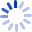# The Analysis of Variance

Originally published in 1959, this classic volume has had a major impact on generations of statisticians. Newly issued in the Wiley Classics Series, the book examines the basic theory of analysis of variance by considering several different mathematical models. Part I looks at the theory of fixed-effects models with independent observations of equal variance, while Part II begins to explore the analysis of variance in the case of other models.

##### Cash for Textbooks